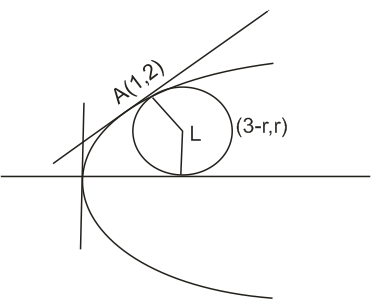Q

# How to solve this problem- - Co-ordinate geometry - JEE Main-9

The area  ( in sq. units ) of the smaller of the two circle that touch the parabola ,  at the point (1,2 ) and the x-axis is :

• Option 1)

• Option 2)

• Option 3)

• Option 4)

Viewsequation of tangent at (1,2)

equation of normal

let the centre be

for

so

Option 1)

Option 2)

Option 3)

Option 4)

Exams
Articles
Questions